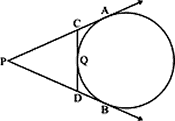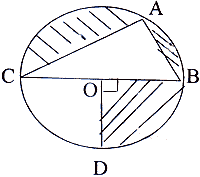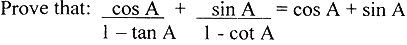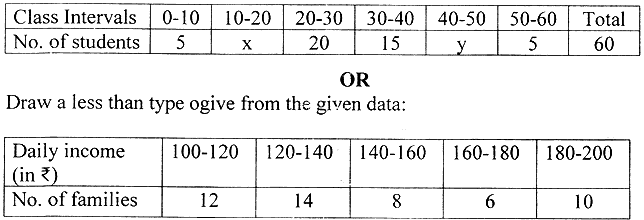Friday , October 23 2020# 10 Class Maths Periodic Test III (2018-19)

## 10th Mathematics Periodic Test III (2018-19)

School Name: Venkateshwar Global School, Sector 13, Rohini, Delhi 110085 India
Time: 3 hours
M.M: 80
Subject Code: 041
Class: X
Subject: Mathematics
Date: 21.01.2019

## General Instructions:

• All questions are compulsory.
• The question paper consist of 30 questions divided into four section A, B, C, and D. Section A comprise of 6 questions of 1 mark each, Section B comprises of 6 questions of 2 marks each, Section C comprises of 10 questions of of 3 marks each and Section D comprises of 8 questions of 4 marks each.
• Use of calculator is not permitted.

## Section A

#### Question: 6. In the given figure, PA and PB are tangents to the circle. CD is a third tangent touching the circle at Q. If PB = 10 cm and CQ = 2cm, what is the length of PC?## Section C

#### Question: 15. Size of agriculture holding in a survey of 200 families is given in the following table:Computer the mode size of holding.

#### Question: 21. In the adjoining figure, O is the centre of a circle with diameter 25 cm. Also, AC = 24cm, AB = 7cm and ∠BOD = 90°. Find the area of the shaded region. (use π = 3. 14)## Section D

#### Question: 24.#### Question: 26. If the median of the distribution given below is 28.5, find the value of x and y.## 9th CBSE Social Studies Examination: Mid Term 2019

9th CBSE Social Studies Examination: Mid Examination Question Paper (2019-20) School Name: Himalaya Public School, Sector …